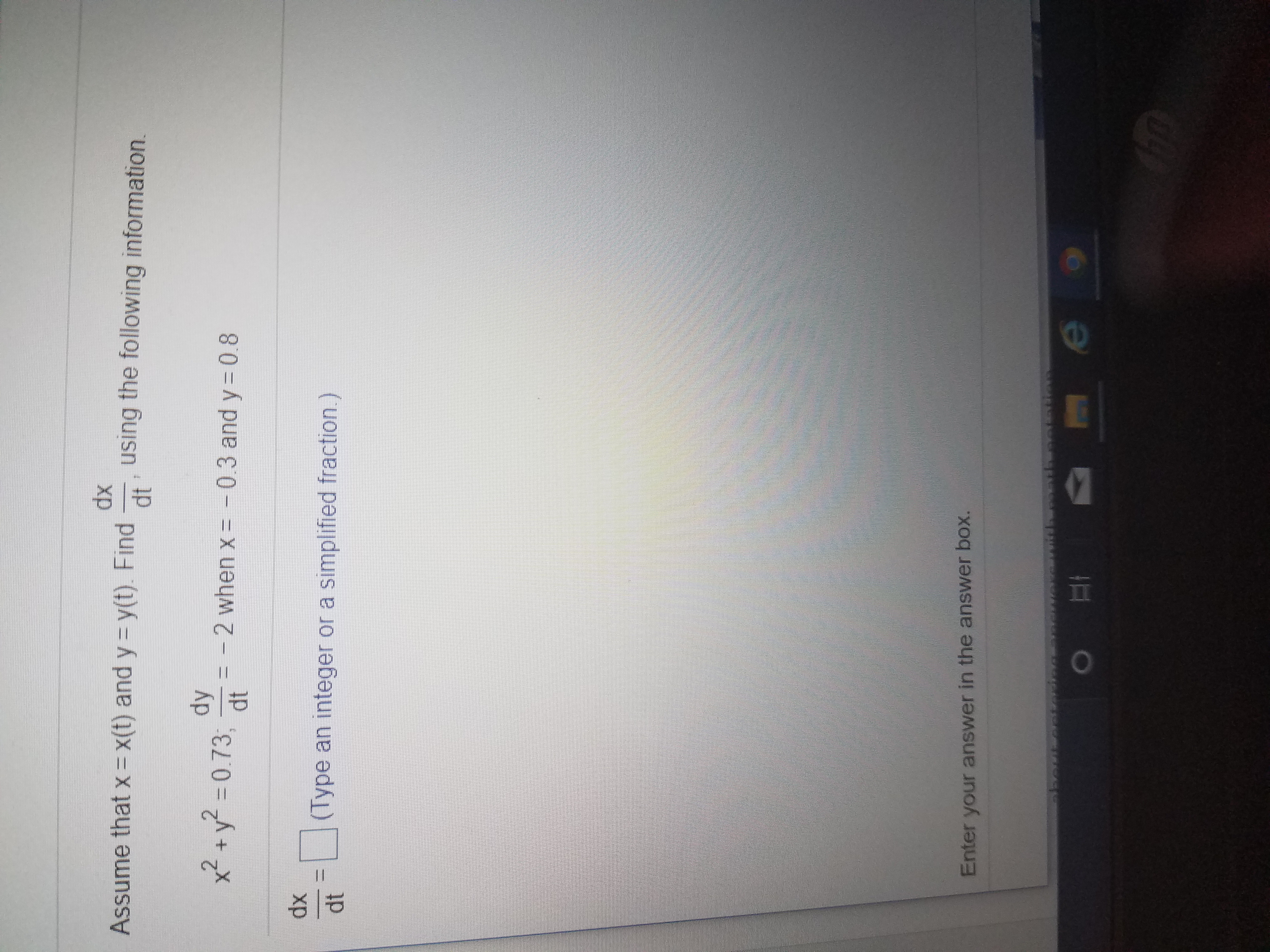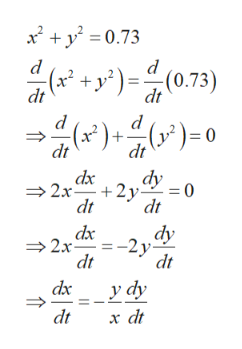# dxAssume that x = x(t) and y y(t). Findusing the following information.dtdy2+y0.73,- 2 when xdt-0.3 and y 0.8dx(Type an integer or a simplified fraction.)dtEnter your answer in the answer box.eO

Questionhelp_outlineImage Transcriptionclosedx Assume that x = x(t) and y y(t). Find using the following information. dt dy 2+y0.73, - 2 when x dt -0.3 and y 0.8 dx (Type an integer or a simplified fraction.) dt Enter your answer in the answer box. e O fullscreen
check_circleExpert Solution
Step 1

Differentiate both sides of the g...help_outlineImage Transcriptionclose2y0.73 (x2 di( +y?)=(0.73) dt d (x2)+ (y)= dt dt dx 2x 2. dt 0= dt dy 2x -2y dt dx dt у ду dt x dt fullscreen

### Want to see the full answer?

See Solution

#### Want to see this answer and more?

Solutions are written by subject experts who are available 24/7. Questions are typically answered within 1 hour*

See Solution
*Response times may vary by subject and question
Tagged in

### Calculus